# pulp: Pulp classes¶

Todo

LpFractionConstraint, FractionElasticSubProblem

## The LpProblem Class¶

class pulp.LpProblem(name='NoName', sense=1)

Bases: object

An LP Problem

Creates an LP Problem

This function creates a new LP Problem with the specified associated parameters

Parameters: name – name of the problem used in the output .lp file sense – of the LP problem objective. Either LpMinimize (default) or LpMaximize. An LP Problem

Three important attributes of the problem are:

objective
The objective of the problem, an LpAffineExpression
constraints
An ordered dictionary of constraints of the problem - indexed by their names.
status
The return status of the problem from the solver.

Some of the more important methods:

solve(solver=None, **kwargs)

Solve the given Lp problem.

This function changes the problem to make it suitable for solving then calls the solver.actualSolve method to find the solution

Parameter: solver – Optional: the specific solver to be used, defaults to the default solver.
Side Effects:
• The attributes of the problem object are changed in actualSolve() to reflect the Lp solution
roundSolution(epsInt=1.0000000000000001e-05, eps=9.9999999999999995e-08)

Rounds the lp variables

Inputs:
• none
Side Effects:
• The lp variables are rounded
setObjective(obj)

Sets the input variable as the objective function. Used in Columnwise Modelling

Parameter: obj – the objective function of type LpConstraintVar
Side Effects:
• The objective function is set
writeLP(filename, writeSOS=1, mip=1)

Write the given Lp problem to a .lp file.

This function writes the specifications (objective function, constraints, variables) of the defined Lp problem to a file.

Parameter: filename – the name of the file to be created.
Side Effects:
• The file is created.

## Variables and Expressions¶

class pulp.LpElement(name)
Base class for LpVariable and LpConstraintVar

class pulp.LpVariable(name, lowBound=None, upBound=None, cat='Continuous', e=None)

This class models an LP Variable with the specified associated parameters

Parameters: name – The name of the variable used in the output .lp file lowbound – The lower bound on this variable’s range. Default is negative infinity upBound – The upper bound on this variable’s range. Default is positive infinity cat – The category this variable is in, Integer, Binary or Continuous(default) e – Used for column based modelling: relates to the variable’s existence in the objective function and constraints
adds a variable to the constraints indicated by the LpConstraintVars in e
classmethod dicts(name, indexs, lowBound=None, upBound=None, cat=0, indexStart=[])

Creates a dictionary of LP variables

This function creates a dictionary of LP Variables with the specified
associated parameters.
Parameters: name – The prefix to the name of each LP variable created indexs – A list of strings of the keys to the dictionary of LP variables, and the main part of the variable name itself lowbound – The lower bound on these variables’ range. Default is negative infinity upBound – The upper bound on these variables’ range. Default is positive infinity cat – The category these variables are in, Integer or Continuous(default) A dictionary of LP Variables
setInitialValue(val)
sets the initial value of the Variable to val may of may not be supported by the solver

Example:

>>> x = LpVariable('x',lowBound = 0, cat='Continuous')
>>> y = LpVariable('y', upBound = 5, cat='Integer')


gives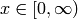,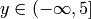, an integer.

class pulp.LpAffineExpression(e=None, constant=0, name=None)

Bases: pulp.odict.OrderedDict

A linear combination of LpVariables. Can be initialised with the following:

1. e = None: an empty Expression
2. e = dict: gives an expression with the values being the coefficients of the keys (order of terms is undetermined)
3. e = list or generator of 2-tuples: equivalent to dict.items()
4. e = LpElement: an expression of length 1 with the coefficient 1
5. e = other: the constant is initialised as e

Examples:

>>> f=LpAffineExpression(LpElement('x'))
>>> f
1*x + 0
>>> d = LpAffineExpression(dict(x_0=1, x_1=-3, x_2=4))
>>> d
1*x_0 + -3*x_1 + 4*x_2 + 0
>>> x_name = ['x_0', 'x_1', 'x_2']
>>> x = [LpVariable(x_name[i], lowBound = 0, upBound = 10) for i in range(3) ]
>>> c = LpAffineExpression([ (x,1), (x,-3), (x,4)])
>>> c
1*x_0 + -3*x_1 + 4*x_2 + 0
>>> c == d
1*x_0 + -3*x_1 + 4*x_2 + -1*x_0 + 3*x_1 + -4*x_2 + 0 = 0
>>> ( c == d) == LpConstraint(0)
<class 'pulp.pulp.LpConstraint'>


In brief,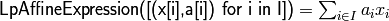where (note the order):

• x[i] is an LpVariable
• a[i] is a numerical coefficient.

pulp.lpSum(vector)

Calculate the sum of a list of linear expressions

Parameter: vector – A list of linear expressions

## Constraints¶

class pulp.LpConstraint(e=None, sense=0, name=None, rhs=None)

Bases: pulp.pulp.LpAffineExpression

An LP constraint

Parameters: e – an instance of LpAffineExpression sense – one of LpConstraintEQ, LpConstraintGE, LpConstraintLE (0, 1, -1 respectively) name – identifying string rhs – numerical value of constraint target
makeElasticSubProblem(*args, **kwargs)

Builds an elastic subproblem by adding variables to a hard constraint

uses FixedElasticSubProblem

### Elastic Constraints¶

A constraint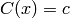(equality may be replaced by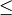or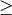) can be elasticized to the form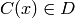where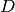denotes some interval containing the value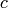.

Define the constraint in two steps:

1. instantiate constraint (subclass of LpConstraint) with target.
2. call its makeElasticSubProblem() method which returns an object of type FixedElasticSubProblem (subclass of LpProblem) - its objective is the minimization of the distance of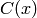from.
constraint = LpConstraint(..., rhs = c)
elasticProblem = constraint.makeElasticSubProblem(
penalty = <penalty_value>,
proportionFreeBound = <freebound_value>,
proportionFreeBoundList = <freebound_list_value>,
)
where:
• <penalty_value> is a real number
• <freebound_value>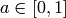specifies a symmetric target interval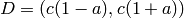about• <freebound_list_value> = [a,b], a list of proportions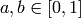specifying an asymmetric target interval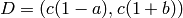aboutThe penalty applies to the constraint at points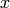where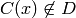. The magnitude of <penalty_value> can be assessed by examining the final objective function in the .lp file written by LpProblem.writeLP().

Example:

>>> constraint_1 = LpConstraint('ex_1',sense=1,rhs=200)
>>> elasticProblem_1 = constraint_1.makeElasticSubproblem(penalty=1, proportionFreeBound = 0.01)
>>> constraint_2 = LpConstraint('ex_2',sense=0,rhs=500)
>>> elasticProblem_2 = constraint_2.makeElasticSubproblem(penalty=1,
proportionFreeBoundList = [0.02, 0.05])

1. constraint_1 has a penalty-free target interval of 1% either side of the rhs value, 200
2. constraint_2 has a penalty-free target interval of - 2% on left and 5% on the right side of the rhs value, 500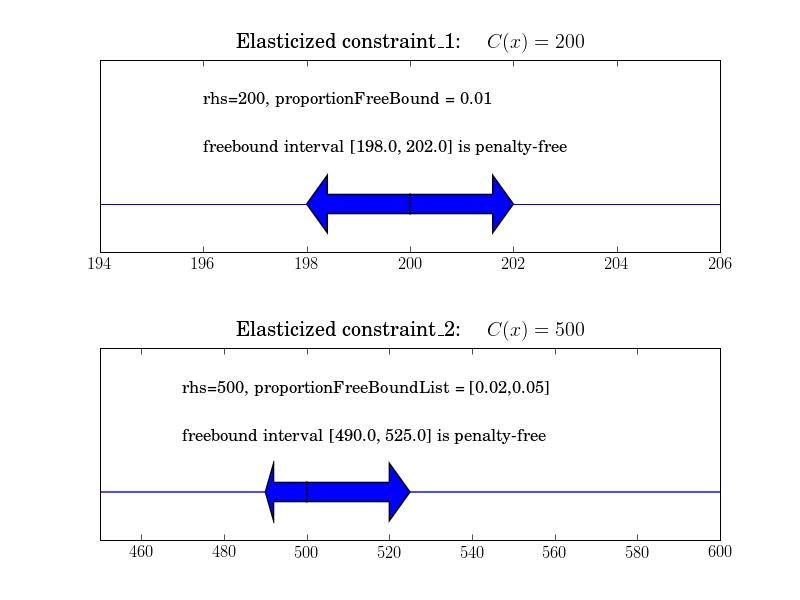Following are the methods of the return-value:

class pulp.FixedElasticSubProblem(constraint, penalty=None, proportionFreeBound=None, proportionFreeBoundList=None)

Bases: pulp.pulp.LpProblem

Contains the subproblem generated by converting a fixed constraint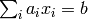into an elastic constraint.

Parameters: constraint – The LpConstraint that the elastic constraint is based on penalty – penalty applied for violation (+ve or -ve) of the constraints proportionFreeBound – the proportional bound (+ve and -ve) on constraint violation that is free from penalty proportionFreeBoundList – the proportional bound on constraint violation that is free from penalty, expressed as a list where [-ve, +ve]
alterName(name)
Alters the name of anonymous parts of the problem
findDifferenceFromRHS()
The amount the actual value varies from the RHS (sense: LHS - RHS)
findLHSValue()
finds the LHS value of the constraint (without the free variable and/or penalty variable) - assumes the constant is on the rhs
isViolated()
returns true if the penalty variables are non-zero

## Combinations and Permutations¶

pulp.combination(orgset, k=None)

returns an iterator that lists the combinations of orgset of length k

Parameters: orgset – the list to be iterated k – the cardinality of the subsets an iterator of the subsets

example:

>>> c = combination([1,2,3,4],2)
>>> for s in c:
...     print s
(1, 2)
(1, 3)
(1, 4)
(2, 3)
(2, 4)
(3, 4)

pulp.allcombinations(orgset, k)

returns all permutations of orgset with up to k items

Parameters: orgset – the list to be iterated k – the maxcardinality of the subsets an iterator of the subsets

example:

>>> c = allcombinations([1,2,3,4],2)
>>> for s in c:
...     print s
(1,)
(2,)
(3,)
(4,)
(1, 2)
(1, 3)
(1, 4)
(2, 3)
(2, 4)
(3, 4)

pulp.permutation(orgset, k=None)

returns an iterator that lists the permutations of orgset of length k

Parameters: orgset – the list to be iterated k – the cardinality of the subsets an iterator of the subsets

example:

>>> c = permutation([1,2,3,4],2)
>>> for s in c:
...     print s
(1, 2)
(1, 3)
(1, 4)
(2, 1)
(2, 3)
(2, 4)
(3, 1)
(3, 2)
(3, 4)
(4, 1)
(4, 2)
(4, 3)

pulp.allpermutations(orgset, k)

returns all permutations of orgset with up to k items

Parameters: orgset – the list to be iterated k – the maxcardinality of the subsets an iterator of the subsets

example:

>>> c = allpermutations([1,2,3,4],2)
>>> for s in c:
...     print s
(1,)
(2,)
(3,)
(4,)
(1, 2)
(1, 3)
(1, 4)
(2, 1)
(2, 3)
(2, 4)
(3, 1)
(3, 2)
(3, 4)
(4, 1)
(4, 2)
(4, 3)

pulp.value(x)
Returns the value of the variable/expression x, or x if it is a number